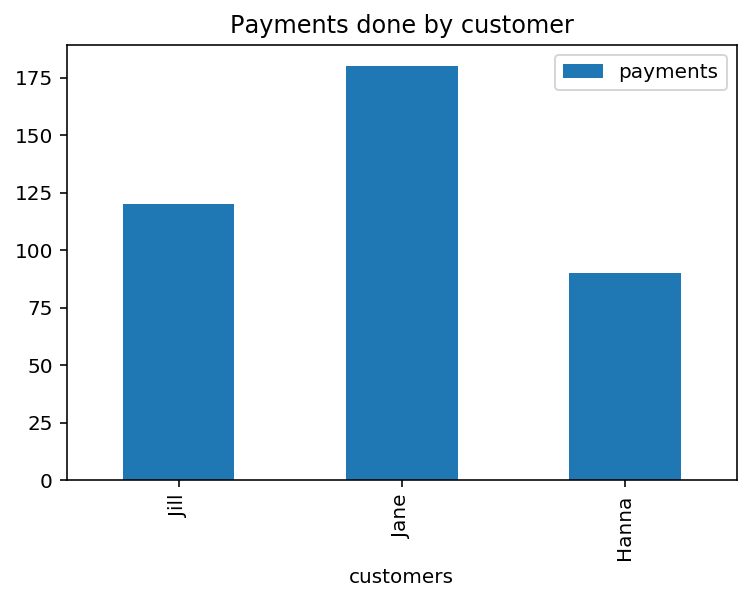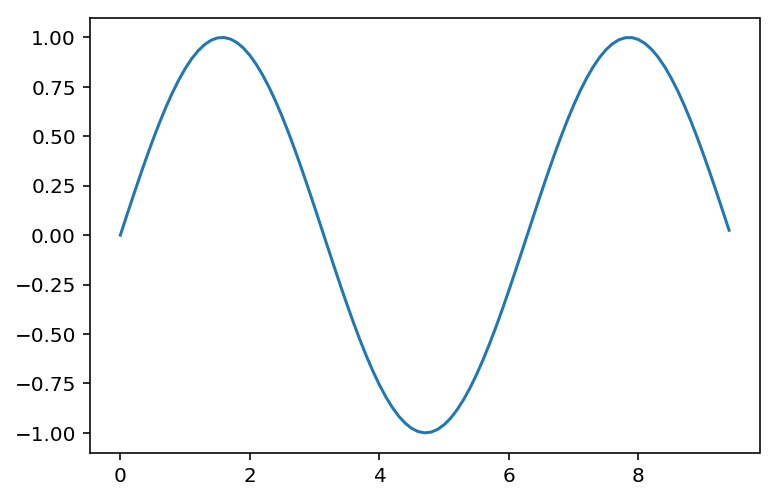# Curiousily

## Machine Learning fundamentals | Machine Learning from Scratch (Part 0)

Machine Learning, Fundamentals, Training, Data Science4 min read

Share

TL;DR Learn about the basics of Machine Learning - what types of learning exist, why implement algorithms from scratch and can you really trust your models?

# .css-kfjr0z{color:var(--theme-ui-colors-primary,#3182ce);-webkit-text-decoration:none;text-decoration:none;}.css-kfjr0z:hover{-webkit-text-decoration:underline;text-decoration:underline;}.css-kfjr0z svg{fill:var(--theme-ui-colors-secondary,#4a5568);margin-right:0.5rem;}Sick of being a lamer?

Here’s a definition for “lamer” from urban dictionary:

Lamer is someone who thinks they are smart, yet are really a loser. E.g. that hacker wannabe is really just a lamer.

You might’ve noticed that reading through Deep Learning and TensorFlow/PyTorch tutorials might give you an idea of how to do a specific task, but fall short if you want to apply it to your own problems. Importing a library and calling 4-5 methods might get the job done but leave you clueless about why it works. Can you solve this problem?

“What I cannot create, I do not understand” - Richard Feynman

Different people learn in different styles, but all hackers learn the same way - we build stuff!

## How these series help?

• Provide a clear path to learning (reduce choice overload and paralysis by analysis) increasingly complex Machine Learning models
• Succint implementations of Machine Learning algorithms solving real-world problems you can thinker with
• Just enough theory + math that helps you understand why things work after you understand what problem you have to solve

# What is Machine Learning?

Machine Learning (ML) is the art and science of teaching machines to do complex tasks without explicitly programming them. Your job, as a hacker, is to:

• Define the problem in a way that a computer can understand it
• Choose a set of possible models that could solve it
• Feed the data
• Evaluate the performance and improve

There are 3 main types of learning: supervised, unsupervised and reinforcement

## Supervised Learning

In Supervised Learning setting, you have a dataset, which is a collection of $N$ labeled examples. Each example has a vector of features $x_i$ and a label $y_i$. The label $y_i$ can belong to a finite set of classes $\{1, \ldots, C\}$, real number or something more complex.

The goal of supervised learning algorithms is to build a model that receives a feature vector $x$ as input and infer the correct label for it.

## Unsupervised Learning

In Unsupervised Learning setting, you have a dataset of $N$ unlabeled examples. Again, you have a feature vector $x$, and the goal is to build a model that takes it and transforms it into another. Some practical examples include clustering, reducing the number of dimensions and anomaly detection.

## Reinforcement Learning

Reinforcement Learning is concerned with building agents that interact with an environment by getting its state and executing an action. Actions provide rewards and change the state of the environment. The goal is to learn a set of actions that maximize the total reward.

# What Learning Algorithms are made of?

Each learning algorithm we’re going to have a look at consists of three parts

• loss function - measure of how wrong your model currently is
• optimization criteria based on the loss function
• optimization routine that uses data to find “good” solutions according to the optimization criteria

These are the main components of all the algorithms we’re going to implement. While there are many optimization routines, Stochastic Gradient Descent is the most used in practice. It is used to find optimal parameters for logistic regression, neural networks, and many other models.

# Making predictions

How can you guarantee that your model will make correct predictions when deployed in production? Well, only suckers think that this is possible.

“All models are wrong, but some are useful.” - George Box

That said, there are ways to increase the prediction accuracy of your models. If the data used for training were selected randomly, independently of one another and following the same procedure for generating it, then, it is more likely your model to learn better. Still, for situations that are less likely to happen, your model will probably make errors.

Generally, the larger the data set, the better the predictions you can expect.

We’re going to use a lot of libraries provided by different kind people, but the main ones are NumPy, Pandas and Matplotlib.

## NumPy

NumPy is the fundamental package for scientific computing with Python. It contains among other things:

• a powerful N-dimensional array object
• tools for integrating C/C++ and Fortran code
• useful linear algebra, Fourier transform, and random number capabilities

Besides its obvious scientific uses, NumPy can also be used as an efficient multi-dimensional container of generic data. Arbitrary data-types can be defined. This allows NumPy to seamlessly and speedily integrate with a wide variety of databases.

Here’s a super simple walkthrough:

1import numpy as np23a = np.array([1, 2, 3]) # 1D array4type(a)
1numpy.ndarray
1a.shape
1(3,)

We’ve created 1 dimensional array with 3 elements. You can get the first element of the array:

1a
11

and change it:

1a = 523a
1array([5, 2, 3])

Let’s create a 2D array

1b = np.array([[1, 2, 3], [4, 5, 6], [7, 8, 9]]) # 2D array23b.shape
1(3, 3)

We have 3x3 matrix. Let’s select only the first 2 rows:

1b[0:2]
1array([[1, 2, 3],2       [4, 5, 6]])

## Pandas

pandas is an open source, BSD-licensed library providing high-performance, easy-to-use data structures and data analysis tools for the Python programming language.

We’re going to use pandas as a holder for our datasets. The primary entity we’ll be working with is the DataFrame. We’ll also do some transformations/preprocessing and visualizations.

Let’s start by creating a DataFrame:

1import pandas as pd23df =  pd.DataFrame(dict(4  customers=["Jill", "Jane", "Hanna"],5  payments=[120, 180, 90]6))78df
customerspayments
Jill120
Jane180
Hanna90

You can check the size of the data frame:

1df.shape
1(3, 2)

We have 3 rows and 2 columns. You can use head() to render a preview of the first five rows:

1df.head()
customerspayments
Jill120
Jane180
Hanna90

Let’s check for missing values:

1df.isnull()
customerspayments
FalseFalse
FalseFalse
FalseFalse

You can apply functions such as [sum(https://pandas.pydata.org/pandas-docs/stable/reference/api/pandas.DataFrame.sum.html)] to columns like payments:

1df.payments.sum()
1390

You can even show some charts using plot():

1df.plot(2    kind='bar',3    x='customers',4    y='payments',5    title='Payments done by customer'6);## Matplotlib

Matplotlib is a Python 2D plotting library which produces publication quality figures in a variety of hardcopy formats and interactive environments across platforms.

Here is a quick sample of it:

1import matplotlib.pyplot as plt23x = np.arange(0, 3 * np.pi, 0.1)4y = np.sin(x)56plt.plot(x, y);